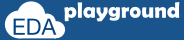# SystemC Operators

### Arithmetic Operators

Arithmetic operators are used to perform arithmetic operation on numbers.
 Operator Description + Addition Add the two operands. – Subtraction Subtract the second operand from the first operand. * Multiplication Multiply the two operands. / Division Divide the first operand by the second operand. % Modulus Modulo operation.

```#include "systemc.h"
int sc_main (int argc, char* argv[]) {
int a = 10;
int b = 20;
int c;

c = a + b;

cout <<"Value of c = " << c << endl;
return 0;// Terminate simulation
}
```

Value of c = 30

### Increment and Decrement

 Operator Description ++ Increment Increment the operand by one and store the result in the operand. — Decrement Decrement the operand by one and store the result in the operand.
Pre Increment and Pre Decrement:
In Pre Increment/Decrement, operator is specified before the operand.

The pre increment/decrement performs the operation first and returns the new value.

Post Increment and Post Decrement:
In Post Increment/Decrement, operator is specified after the operand.
The post increment/decrement  returns the old value while the new value of the operation is stored in the operand.

#### Example-1: Pre-Increment

```#include "systemc.h"
int sc_main (int argc, char* argv[]) {
int a = 5;

//pre-increment
cout <<"Value of a = " << ++a << endl;
return 0;// Terminate simulation
}
```

#### Example-2: Post-Increment

```#include "systemc.h"
int sc_main (int argc, char* argv[]) {
int a = 5;

cout <<"Value of a = " << a++ << endl;
return 0;// Terminate simulation
}
```

### Bitwise Operators

 Operator Description & AND operator Calculate the bitwise AND of the two operands. | OR  operator Calculate the bitwise OR of the two operands. ^ XOR operator Calculate the bitwise XOR of the two operands.

#### Example-1: Bitwise AND Operator

```#include "systemc.h"
int sc_main (int argc, char* argv[]) {
int a = 1;
int b = 1;
int c;

//bitwise AND operator,
c = a & b;

cout <<"Value of c = " << c << endl;
return 0;// Terminate simulation
}
```

Value of c = 1

### Arithmetic and Bitwise assignment

 Operator Description += Add the two operands; assign the result to the first operand. -= Subtract the second operand from the first operand; assign the result to the first operand. *= Multiply the two operands; assign the result to the first operand. /= Divide the first operand by the second operand; assign the result to the first operand. %= Modulo operation; assign the result to the first operand. &= Calculate the bitwise AND of the two operands; assign the result to the first operand. |= Calculate the bitwise OR of the two operands; assign the result to the first operand. ^= Calculate the bitwise XOR of the two operands; assign the result to the first operand.

#### Example-1: += Operator

```#include "systemc.h"
int sc_main (int argc, char* argv[]) {
int a = 10;
int b = 20;

//+= operator, Arithmetic and bitwise assignment
a += b;

cout <<"Value of a = " << a << endl;
return 0;// Terminate simulation
}
```

#### Simulator Output:

Value of a = 30
Execute the above code on#### Example-2: &= Operator

```#include "systemc.h"
int sc_main (int argc, char* argv[]) {
int a = 1;
int b = 0;

//&= operator, Arithmetic and bitwise assignment
a &= b;

cout <<"Value of a = " << a << endl;
return 0;// Terminate simulation
}
```

#### Simulator Output:

Value of a = 0

Execute the above code on### Equality and Relation

 Operator Description == equal Return true if the operands are equal. != not equal Return true if the operands are not equal. < less than Return true if the first operand is less than the second operand. <= less than or equal to Return true if the first operand is less than or equal to the second operand. > greater than Return true if the first operand is greater than the second operand. >= greater than or equal to Return true if the first operand is greater than or equal to the second operand.

#### Example-1: == Operator

```#include "systemc.h"
int sc_main (int argc, char* argv[]) {
int a = 10;
int b = 20;

// == operator, Equality and relation
if(a == b)
cout <<"a is equals to b"<< endl;
else
cout <<"a is not equals to b"<< endl;
return 0;// Terminate simulation
}
```

#### Simulator Output:

a is not equals to b

Execute the above code on